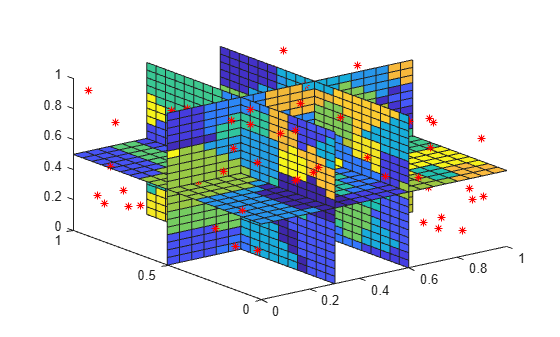# griddatan

Interpolate N-D scattered data

## Syntax

``vq = griddatan(x,v,xq)``
``vq = griddatan(x,v,xq,method)``
``vq = griddatan(x,v,xq,method,options)``

## Description

example

````vq = griddatan(x,v,xq)` fits a hypersurface of the form v = f(x) to the sample points `x` with values `v`. The `griddatan` function interpolates the surface at the query points specified by `xq` and returns the interpolated values, `vq`. The surface always passes through the data points defined by `x` and `v`.```

example

````vq = griddatan(x,v,xq,method)` specifies the interpolation method used to compute `vq`. Options are `'linear'` or `'nearest'`.```
````vq = griddatan(x,v,xq,method,options)` specifies a cell array of character vectors, `options`, to be used in Qhull via `delaunayn`.```

## Examples

collapse all

Interpolate a 4-D scattered data set and visualize a 3-D isosurface of the interpolated data.

Create a scattered set of sample points.

```X = 2*gallery('uniformdata',[5000 3],0)-1; Y = sum(X.^2,2);```

Create x, y, and z grids to use as the 3-D set of query points, and interpolate the scattered data at these points.

```d = -0.8:0.05:0.8; [y0,x0,z0] = ndgrid(d,d,d); XI = [x0(:) y0(:) z0(:)]; YI = griddatan(X,Y,XI);```

Since it is difficult to visualize 4-D data sets, use isosurface at 0.8 to visualize the interpolation result.

```YI = reshape(YI, size(x0)); p = patch(isosurface(x0,y0,z0,YI,0.8)); isonormals(x0,y0,z0,YI,p) p.FaceColor = 'blue'; p.EdgeColor = 'none'; view(3) axis equal camlight lighting phong```Use nearest neighbor interpolation on a 3-D data set.

Create a sample 3-D data set. The matrix `X` contains the xyz locations of the observed data, and `v` contains the (randomly generated) observed data. This type of data set could represent, for example, oxygen levels in ocean water at these locations.

```X = [rand(100,1) rand(100,1) rand(100,1)]; v = rand(100,1);```

Use nearest neighbor interpolation to approximate the value of the underlying function at some query points.

```[xx,yy,zz] = meshgrid(0.2:0.025:0.8); xq = [xx(:) yy(:) zz(:)]; vq = griddatan(X,v,xq,'nearest');```

Plot slices of the result on top of the sample point locations.

```vq = reshape(vq,size(xx)); plot3(X(:,1),X(:,2),X(:,3),'r*') hold on slice(xx,yy,zz,vq,[0.2 0.4 0.6 0.8],0.5,0.5)```## Input Arguments

collapse all

Sample point coordinates, specified as a matrix. Specify `x` as an `m`-by-`n` matrix to represent `m` points in `n`-dimensional space. The sample points must be unique.

Data Types: `single` | `double`

Sample values, specified as a vector. Specify `v` as a vector of length `m`, with one value for each sample point (row) specified in `x`.

If `v` contains complex numbers, then `griddatan` interpolates the real and imaginary parts separately.

Data Types: `single` | `double`
Complex Number Support: Yes

Query points, specified as a matrix. Specify `xq` as a `p`-by-`n` matrix to represent `p` points in `n`-dimensional space. `xq` is usually created from a uniform grid produced by `ndgrid`.

Data Types: `single` | `double`

Interpolation method, specified as one of the values in this table. These methods are both based on a Delaunay triangulation of the input data.

OptionDescriptionContinuity
`'linear'` (default)Triangulation-based linear interpolation.C0
`'nearest'`Nearest neighbor interpolation.Discontinuous

If `method` is `[]`, then `griddatan` uses the default `'linear'` method.

Data Types: `char`

Qhull-specific options, specified as a cell array. For a list of supported options, see Qhull Quick Reference.

If `options` is `[]`, then `griddatan` uses the default options:

• `{'Qt' 'Qbb' 'Qc'}` for 2-D and 3-D interpolations.

• `{'Qt' 'Qbb' 'Qc' 'Qx'}` for interpolations in 4+ dimensions.

If `options` is `{''}`, then `griddatan` does not use any options, not even the defaults.

Data Types: `cell`

## Output Arguments

collapse all

Interpolated values, returned as a vector of length `p`. The interpolated values in `vq` correspond to the query points (rows) in `xq`.

## Tips

• It is not practical to use `griddatan` for interpolation in dimensions higher than about 6-D, because the memory required by the underlying triangulation grows exponentially with the number of dimensions.

• Scattered data interpolation with `griddatan` uses a Delaunay triangulation of the data, so can be sensitive to scaling issues in `x`. When this occurs, you can use `normalize` to rescale the data and improve the results. See Normalize Data with Differing Magnitudes for more information.